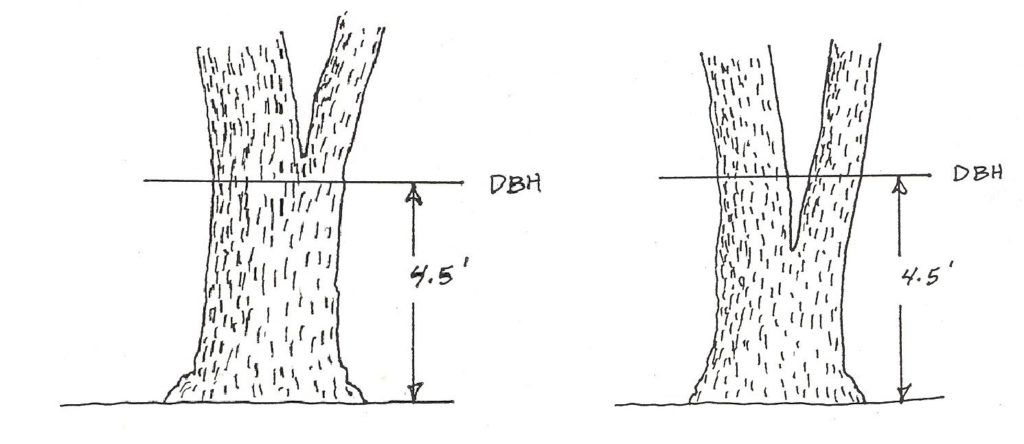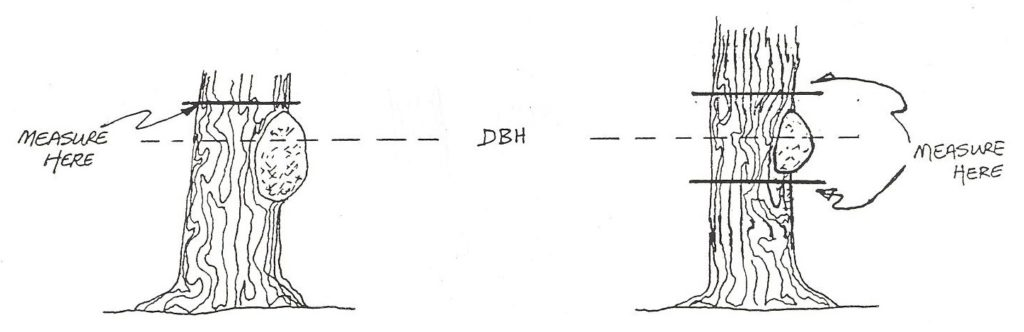# 3.2: Determining Tree Diameter

$$\newcommand{\vecs}{\overset { \rightharpoonup} {\mathbf{#1}} }$$ $$\newcommand{\vecd}{\overset{-\!-\!\rightharpoonup}{\vphantom{a}\smash {#1}}}$$$$\newcommand{\id}{\mathrm{id}}$$ $$\newcommand{\Span}{\mathrm{span}}$$ $$\newcommand{\kernel}{\mathrm{null}\,}$$ $$\newcommand{\range}{\mathrm{range}\,}$$ $$\newcommand{\RealPart}{\mathrm{Re}}$$ $$\newcommand{\ImaginaryPart}{\mathrm{Im}}$$ $$\newcommand{\Argument}{\mathrm{Arg}}$$ $$\newcommand{\norm}{\| #1 \|}$$ $$\newcommand{\inner}{\langle #1, #2 \rangle}$$ $$\newcommand{\Span}{\mathrm{span}}$$ $$\newcommand{\id}{\mathrm{id}}$$ $$\newcommand{\Span}{\mathrm{span}}$$ $$\newcommand{\kernel}{\mathrm{null}\,}$$ $$\newcommand{\range}{\mathrm{range}\,}$$ $$\newcommand{\RealPart}{\mathrm{Re}}$$ $$\newcommand{\ImaginaryPart}{\mathrm{Im}}$$ $$\newcommand{\Argument}{\mathrm{Arg}}$$ $$\newcommand{\norm}{\| #1 \|}$$ $$\newcommand{\inner}{\langle #1, #2 \rangle}$$ $$\newcommand{\Span}{\mathrm{span}}$$$$\newcommand{\AA}{\unicode[.8,0]{x212B}}$$

## 3.2 Determining Tree DiameterFigure 3.2. A tree showing how diameters are not constant as one moves up a tree.

Trees do not grow like cylinders, but rather taper upward, the tree’s diameter getting smaller as one gets closer to the top of the tree. Trees also have butt swell, a thickening of the wood and bark at the base of the tree to support the tree’s mass (Figure 3.2). Butt swell can create a very large diameter on trees exposed to heavy wind, on steep slopes and in sparsely populated stands. Thus, when you really think about trying to obtain useful diameter data, the question becomes, “where on the tree should I measure it?”

To make tree diameter measurements meaningful and easy to perform, a standard location and protocol have been developed. Diameters are measured outside the bark at Diameter at Breast Height (DBH), or 4½ feet above the ground on the uphill side of the tree (Figure 3.3). This location is above most butt swell, above most of the brush, and is at a comfortable arm position for most people.Figure 3.3. The standard location for measuring tree diameter is at DBH, 4.5 feet above the ground on the uphill side of the tree. (From [FS] 1990.)

For most trees in the forest, measuring dbh is quite straightforward. However, there are plenty of irregular trees that require adaptations (Figures 3.4 – 3.10). (All illustrations from or adapted from [FS] 1990.)Figure 3.4. On forked trees, measure as one tree if fork occurs at or above 4.5’ (left). Measure as two trees if fork occurs below 4.5’ (right).Figure 3.5. Measure directly above a bulge or branch whorl (left). On trees with extensive butt swell, measure at least 1½’ above the butt swell (right).Figure 3.6. For a large burl or canker, measure above the deformity and adjust the diameter down slightly (left), or take two measures equidistant from dbh above and below the deformity, and use the average (right).Figure 3.7. On leaning trees, the tape is held perpendicular to the tree bole, and is measured on the uphill side of the tree if on a slope (left); on the short side of the lean if on flat ground (right).Figure 3.8. On trees with roots above ground, measure at 4.5’ above the root crown (below).Figure 3.9. On trees that have grown together, count as two trees. Measure halfway around each, and double the measurement.Figure 3.10. On trees with scars, treat as a double tree if severe (left), or reconstruct the diameter if slight (right).

This page titled 3.2: Determining Tree Diameter is shared under a CC BY license and was authored, remixed, and/or curated by Joan DeYoung (OpenOregon) .